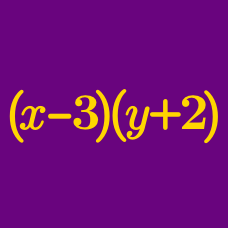Algebra

# Applying the Perfect Square Identity

If $x^2+20xy+100y^2=(x+ay)^2,$ what is the value of $$a$$?

If $x^2+121-(x-a)^2=22x,$ what is the value of $$a$$?

If $16x^2+56xy+49y^2=(ax+by)^2,$ what is the value of $$ab$$?

Given $a-b=\sqrt{42+12\sqrt{6}}, b-c=\sqrt{42-12\sqrt{6}},$ what is the value of $a^2+b^2+c^2-ab-bc-ca?$

What is the coefficient of $$x$$ in the expansion of $$(x+3 ) ^ 2$$?

×